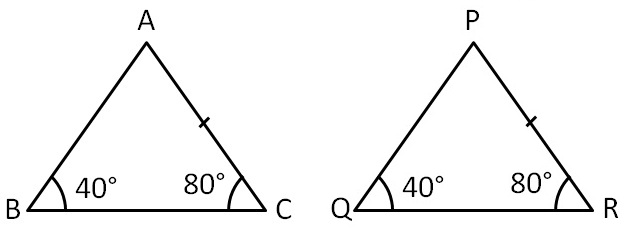ASA Congruency Criteria

Congruence of Triangles
Concept wise

To prove two triangles congruent,

We can also use AAS criteria – Angle Angle Side

This criteria is equivalent to ASA Criteria.

Here’s how:

Suppose we are given two triangles Δ ABC & Δ PQRLet’s prove this by ASA congruency finding ∠A & ∠P

 In ∆ABC, In ∆PQR , By Angle Sum Property, ∠A + ∠B + ∠C = 180° ∠A + 40° + 80° = 180° ∠A + 120° = 180° ∠A = 180° − 120° ∠A = 60° By Angle Sum Property, ∠P + ∠Q + ∠R = 180° ∠P + 40° + 80° = 180° ∠P + 120° = 180° ∠P = 180° − 120° ∠P = 60°Now, In ∆ABC and ∆PQR,

∠A = ∠P           (Both are 60°)

AC = PR           (Given )

∠C = ∠R           (Both are 80°)

∴ ∆ABC ≅ ∆PQR     (ASA congruence rule)

OR

We can prove this by AAS

In ∆ABC and ∆PQR

∠B = ∠Q        (Both are 40°)

∠C = ∠R        (Both are 80°)

AC = PR         ( Given )

∴ ∆ABC ≅ ∆PQR      (AAS congruence rule)

Learn in your speed, with individual attention - Teachoo Maths 1-on-1 Class